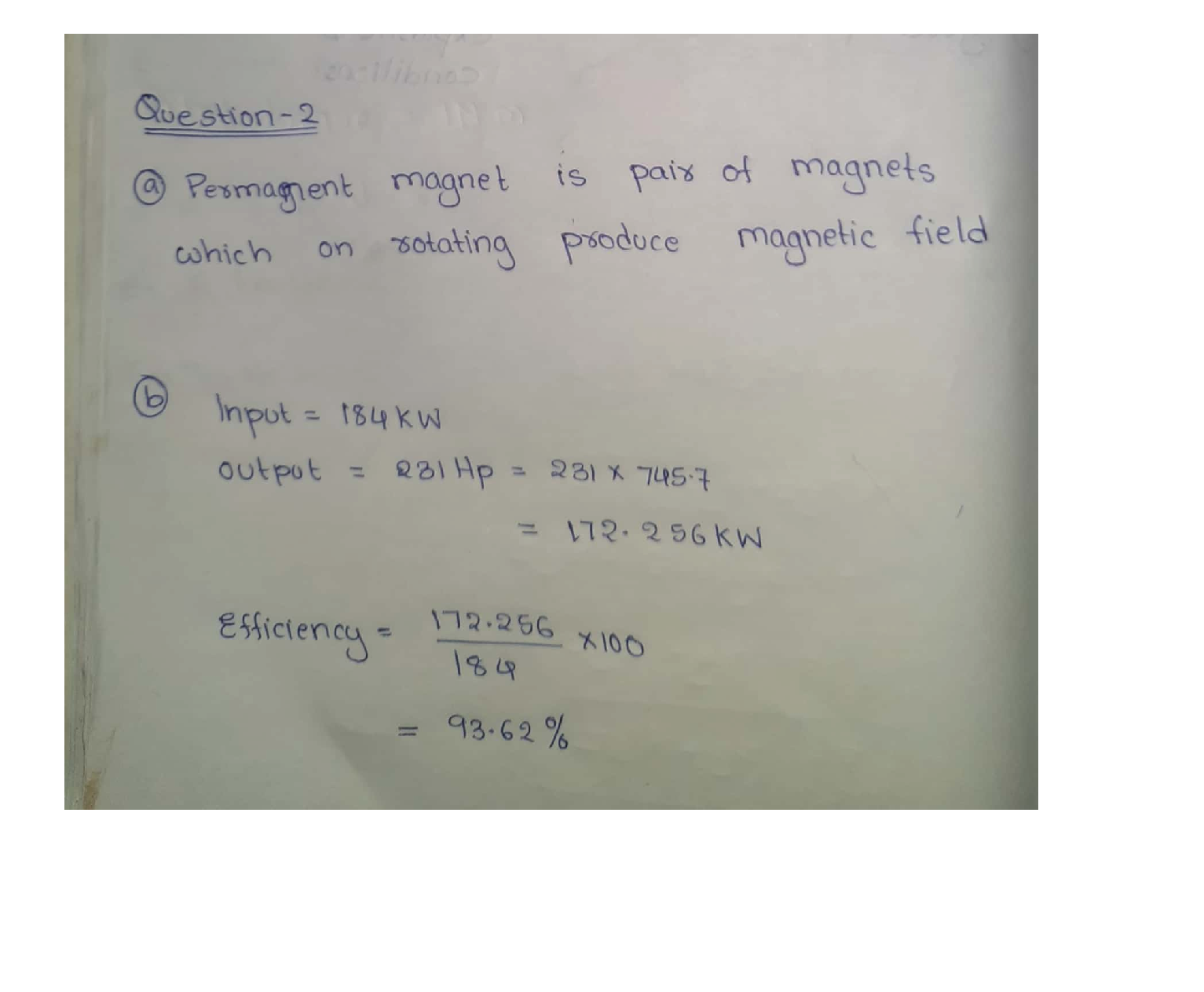Question

Electrical Machines

DC motors use permanent magnets to construct a static magnetic field.

If a motor consumes 184 kW of power but only produces 231 HP at the shaft, its current operating efficiency is answer to the nearest one.% (1 Horsepower = 745.7 Watts). Round your

c) Induction motors use commutators to transmit current to the rotor, thus producing rotor magnetic field.a

A live reading of a motor indicates that it is consuming 16.4 kVA with a Power Factor of 0.76. You also know the current operating efficiency of the motor is 89%. What is the instantaneous power output of the motor? (1 Horsepower = 745.7 Watts).Verified### Question 45030Electrical Machines

a) Sketch circuit diagrams showing field connections for the following self-excited generator d.c.generators. In addition connect the following self-excited generator by drawing the properconnections in the two terminal boxes

### Question 44522Electrical Machines

A 3 phase STAR wound induction motor is supplied with a line voltage of400V at 60HZ. Each phase of the stator has 300 turns and the rotor is of the wound type with 200 turns. The Rotor windings have a resistance of100 and an inductance of 0.1H.
When the motor is stationary determine each of the following:
i The rotor EMF
ii The rotor frequency
The rotor current
- The power factor of the rotor

### Question 44521Electrical Machines

A three phase, 10 pole, delta connected induction motor is supplied at440V, 50 Hz. It draws a current of 32A with a power factor of 0.81lagging. The motor runs at 575 RPM.
Total stator, Rotor copper and mechanical losses equate to 3, 4 and 7%of the input power. Determine each of the following:
The slip
The efficiency of the motor
The torque developed in the rotor
The shaft output torque.
Sketch and label the motor power flow chart for the motor described in this question. You answer must clearly identify the input power, output power and power losses.

### Question 43379Electrical Machines

(b) A 1:10 step-up ideal transforner is placed at the output of the generator, and a 10:1step-down ideal transformer is placed at the load end of the transmission line, asshown in Figure (b) below.
\text { (i) What is the new load voltage, } V_{\text {load }} \text { ? }
(ii) What is the ratio of the new load voltage magnitude |Vload to the generated voltage magnitude |Vgl? [1 point]
(iii) What are the transmission power losses Ploss of the system in this case?

### Question 43378Electrical Machines

A 2.48-kVms Single-phase generator supplies power to a load through a transmission line.
\text { The load impedance is } Z_{\text {logel }}=100 \angle 36.87^{\circ} \Omega \text {, and the impedance of the transmission line }
\text { is } Z_{\text {int }}=12 \angle 60^{\circ} \Omega \text {. }
If the generator is connected directly to the load as in Figure (a) below:
(i) What is the load voltage-
What is the ratio of the load voltage magnitude |Viond to the generated voltage magnitude |Val? [1 point]
O What are the transmission power losses Ploss of the system? [2

### Question 43377Electrical Machines

(b) A two-legged core made of steel is shown below, together with its magnetization curve. The winding (Ni) on the left leg of the core has 600 turns, and the winding (N2)on the right leg of the core has 200 turns. The coils are wound in the directions shown in the figure. The dimensions of the core are as shown, and the currents are i 0.5 Aand i = 1.00 A.
(i) How much total flux dot is produced in the core by the specified currents?
(ii) What is the relative permeability 4, of the core under these conditions?

### Question 43376Electrical Machines

(a) The Figure below shows a ferromagnetic core whose mean path length is 40 cm, with a small air-gap of 0.05 cm in length. The cross-sectional area of the core is 12 cm2,and the relative permeability ; of the core is 4000. The coil of the wire on the core has 400 turns. Fringing in the air-gap increases the effective cross-sectional area of the air gap by 5%.
(i) Find the total reluctance of the flux path (core plus air gap). [5 points].
(ii) Find the current, i, required to produce a flux density of 0.5 T in the air-gap.

### Question 43375Electrical Machines

\text { A balanced } Y-\Delta \text { system with } V_{a m}=100 \angle 0^{\circ} V_{r m s} \text { positive phase sequence, has a phase }
\text { load impedance } Z_{p}=6-j 12 \Omega \text {, and an impedance } Z_{\text {linc }}=1.0 \Omega \text { in each line. }
(1)Draw the appropriate circuit.
(ii) What is the total input power, Pin, from the source?
(iii) What is the total loss power, Plass, in the lines?
Vhat is the efficiency of the system?

### Question 43076Electrical Machines

Propose two methods to control the torque of a D.C motor.Does it will effecting the material and cost? Write it in about30 words.

### Question 42472Electrical Machines

8 The manufacturer's data sheet for a 26-kV, 750-MVA, 60-Hz, three-phase synchronous generator indicates that it has a synchronous reactance X, =2.04 and a leakage reactance Xal = 0.18, both in per unit on the base. Calculate (a) the synchronous inductance in mH, (b) the armature leakage inductance in mH, and (c) the armature phase inductance La in m Hand per unit

### Submit query